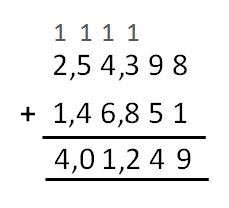Chapter 1 Class 6 Knowing our Numbers
Concept wiseSo, total is 4, 01, 249

Learn in your speed, with individual attention - Teachoo Maths 1-on-1 Class

### Transcript

Adding Large Numbers Suppose we want to add 254398 and 146851 We follow these steps 1. We write the numbers on top of each other (one digit below the other) 2. We start from the right, go towards the left 3. First we add 8 & 1, we get 9. We write 9 below 8 and 1 4. Then we add 9 & 5, we get 14. We write last digit 4 below 9 and 5. and the remaining 1 on top of next digit 3 5. Now, we will add 1, 3 and 8. We get 12. We write last digit 2 below 3 and 8. And write 1 on top of next digit 4. 6. We repeat the same steps to find the answer So, total is 4, 01, 249